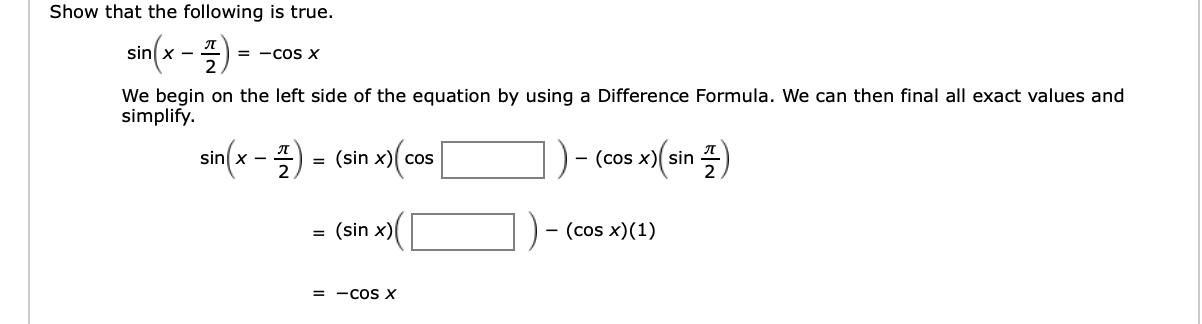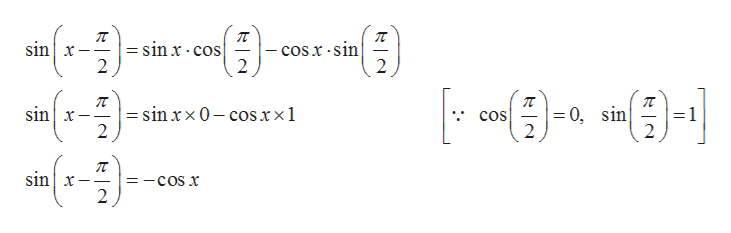# Show that the following is true.sin(x프2= -COS XWe begin on the left side of the equation by using a Difference Formula. We can then final all exact values andsimplifysin(x -2(sin x) cos- (cos x) sin(sin x)- (cos x)(1)= -COS X

Question
1 viewshelp_outlineImage TranscriptioncloseShow that the following is true. sin(x 프2 = -COS X We begin on the left side of the equation by using a Difference Formula. We can then final all exact values and simplify sin(x -2 (sin x) cos - (cos x) sin (sin x) - (cos x)(1) = -COS X fullscreen
check_circle

Step 1

Given:

Step 2

Identity used:

Step 3

According to question starting with...help_outlineImage Transcriptionclosesin x = sinx cos 2 cosx-sin 2 2 sin x 0, sin 2 = sin x x 0- cosxx1 2 -1 2 cos sinx 2 =_ fullscreen

### Want to see the full answer?

See Solution

#### Want to see this answer and more?

Solutions are written by subject experts who are available 24/7. Questions are typically answered within 1 hour.*

See Solution
*Response times may vary by subject and question.
Tagged in

### Trigonometric Ratios